• loss函数之L1Loss，MSELoss，SmoothL1Loss, HuberLoss
千次阅读
2021-06-14 23:10:27

### L1Loss

平均绝对误差（MAE），用于回归模型

对于包含 N N 个样本的batch数据 D ( x , y ) D(x, y) x x 为神经网络的输出， y y 是真实的得分， x x y y 同维度。

n n 个样本的损失值 l n l_{n} 计算如下:

l n = ∣ x n − y n ∣ l_{n}=\left|x_{n}-y_{n}\right|

其中， y n y_{n} 代表第 n n 样本的真实得分，可能对应一个值，也可能多个值，代表样本不同方面的得分，所以 l n l_{n} 可能是一个值，也可能是一个向量。

class L1Loss(_Loss):
def __init__(self, size_average=None, reduce=None, reduction='mean'):
super(L1Loss, self).__init__(size_average, reduce, reduction)
def forward(self, input, target):
return F.l1_loss(input, target, reduction=self.reduction)


pytorch中通过torch.nn.L1Loss类实现，也可以直接调用F.l1_loss 函数，代码中的size_averagereduce已经弃用。reduction有三种取值mean, sum, none，对应不同的返回 ℓ ( x , y ) \ell(x, y) 。 默认为mean，对 L L 中所有元素求平均，对应于一般情况下的 l o s s loss 的计算。

L = { l 1 , … , l N } L=\left\{l_{1}, \ldots, l_{N}\right\}

ℓ ( x , y ) = { L ⁡ ,  if reduction  =  ’none’  mean ⁡ ( L ) ,  if reduction  =  ’mean’  sum ⁡ ( L ) ,  if reduction  =  ’sum’  \ell(x, y)=\left\{\begin{array}{ll}\operatorname L, & \text { if reduction }=\text { 'none' } \\ \operatorname{mean}(L), & \text { if reduction }=\text { 'mean' } \\ \operatorname{sum}(L), & \text { if reduction }=\text { 'sum' }\end{array} \right.

### MSELoss

均方误差（MSE），用于回归模型

对于包含 N N 个样本的batch数据 D ( x , y ) D(x, y) x x 为神经网络的输出， y y 是真实的得分。

n n 个样本的损失值 l n l_{n} 计算如下:

l n = ( x n − y n ) 2 l_{n}=\left(x_{n}-y_{n}\right)^{2}

其中， y n y_{n} 代表第 n n 样本的真实得分，可能对应一个值，也可能多个值，代表样本不同方面的得分，所以 l n l_{n} 可能是一个值，也可能是一个向量。

class MSELoss(_Loss):
def __init__(self, size_average=None, reduce=None, reduction='mean'):
super(MSELoss, self).__init__(size_average, reduce, reduction)
def forward(self, input, target):
return F.mse_loss(input, target, reduction=self.reduction)


pytorch中通过torch.nn.MSELoss类实现，也可以直接调用F.mse_loss 函数。代码中的size_averagereduce已经弃用。reduction有三种取值mean, sum, none，对应不同的返回 ℓ ( x , y ) \ell(x, y) 。默认为mean，对 L L 中所有元素求平均，对应于一般情况下的 l o s s loss 的计算。

L = { l 1 , … , l N } L=\left\{l_{1}, \ldots, l_{N}\right\}

ℓ ( x , y ) = { L ⁡ ,  if reduction  =  ’none’  mean ⁡ ( L ) ,  if reduction  =  ’mean’  sum ⁡ ( L ) ,  if reduction  =  ’sum’  \ell(x, y)=\left\{\begin{array}{ll}\operatorname L, & \text { if reduction }=\text { 'none' } \\ \operatorname{mean}(L), & \text { if reduction }=\text { 'mean' } \\ \operatorname{sum}(L), & \text { if reduction }=\text { 'sum' }\end{array} \right.

### SmoothL1Loss

分段使用均方误差和平均绝对误差，用于回归模型

对于包含 N N 个样本的batch数据 D ( x , y ) D(x, y) x x 为神经网络的输出， y y 是真实的得分。

n n 个样本的损失值 l n l_{n} 计算如下:

l n = { 0.5 ( x n − y n ) 2 /  beta  ,  if  ∣ x n − y n ∣ <  beta  ∣ x n − y n ∣ − 0.5 ∗  beta  ,  otherwise  l_{n}=\left\{\begin{array}{ll}0.5\left(x_{n}-y_{n}\right)^{2} / \text { beta }, & \text { if }\left|x_{n}-y_{n}\right|<\text { beta } \\ \left|x_{n}-y_{n}\right|-0.5 * \text { beta }, & \text { otherwise }\end{array}\right.

其中， y n y_{n} 代表第 n n 样本的真实得分，可能对应一个值，也可能多个值，代表样本不同方面的得分，所以 l n l_{n} 可能是一个值，也可能是一个向量。

相比平均绝对误差，SmoothL1Loss平滑了 ∣ x n − y n ∣ \left|x_{n}-y_{n}\right| 趋近于0时的误差。相比均方误差函数，SmoothL1Loss对离群点更不敏感。在一定程度上可以防止梯度爆炸问题。Fast R-CNN论文有详细论述。

class SmoothL1Loss(_Loss):
def __init__(self, size_average=None, reduce=None, reduction: str = 'mean', beta: float = 1.0) -> None:
super(SmoothL1Loss, self).__init__(size_average, reduce, reduction)
self.beta = beta
def forward(self, input: Tensor, target: Tensor) -> Tensor:
return F.smooth_l1_loss(input, target, reduction=self.reduction, beta=self.beta)


pytorch中通过torch.nn.SmoothL1Loss类实现，也可以直接调用F.smooth_l1_loss 函数。代码中的size_averagereduce已经弃用。reduction有三种取值mean, sum, none，对应不同的返回 ℓ ( x , y ) \ell(x, y) 。默认为mean，对 L L 中所有元素求平均，对应于一般情况下的 l o s s loss 的计算。

L = { l 1 , … , l N } L=\left\{l_{1}, \ldots, l_{N}\right\}

ℓ ( x , y ) = { L ⁡ ,  if reduction  =  ’none’  mean ⁡ ( L ) ,  if reduction  =  ’mean’  sum ⁡ ( L ) ,  if reduction  =  ’sum’  \ell(x, y)=\left\{\begin{array}{ll}\operatorname L, & \text { if reduction }=\text { 'none' } \\ \operatorname{mean}(L), & \text { if reduction }=\text { 'mean' } \\ \operatorname{sum}(L), & \text { if reduction }=\text { 'sum' }\end{array} \right.

参数 b e t a > = 0 beta>=0 ，默认为1

### HuberLoss

分段使用均方误差和平均绝对误差，用于回归模型

对于包含 N N 个样本的batch数据 D ( x , y ) D(x, y) x x 为神经网络的输出， y y 是真实的得分。

n n 个样本的损失值 l n l_{n} 计算如下:

l n = { 0.5 ( x n − y n ) 2 ,  if  ∣ x n − y n ∣ <  beta   beta  ∗ ( ∣ x n − y n ∣ − 0.5 ∗  beta  ) ,  otherwise  l_{n}=\left\{\begin{array}{ll}0.5\left(x_{n}-y_{n}\right)^{2}, & \text { if }\left|x_{n}-y_{n}\right|<\text { beta } \\ \text { beta }*(\left|x_{n}-y_{n}\right|-0.5 * \text { beta }), & \text { otherwise }\end{array}\right.

对比SmoothL1LossHuberLoss公式可知， HuberLoss = beta ∗ SmoothL1Loss \text {HuberLoss}= \text {beta}* \text {SmoothL1Loss} ，两者有如下区别：

• b e t a beta 趋于0时，SmoothL1Loss收敛于L1Loss, HuberLoss收敛于常数0
• b e t a beta 趋于无穷时，SmoothL1Loss收敛于常数0，HuberLoss收敛于MSELoss
• 随着 b e t a beta 的变化，SmoothL1Loss中平均绝对误差段的斜率恒定为1；而HuberLos中平均绝对误差段的斜率是 b e t a beta

SmoothL1Loss 例子：

import torch
import torch.nn as nn
import math

def validate_loss(output, target, beta):
val = 0
for li_x, li_y in zip(output, target):
for i, xy in enumerate(zip(li_x, li_y)):
x, y = xy
if math.fabs(x - y) < beta:
loss_val = 0.5 * math.pow(x - y, 2) / beta
else:
loss_val = math.fabs(x - y) - 0.5 * beta
val += loss_val
return val / output.nelement()

beta = 1
loss_fct = nn.SmoothL1Loss(reduction="mean", beta=beta)
input_src = torch.Tensor([[0.8, 0.8], [0.9, 0.9], [0.3, 0.3]])
target = torch.Tensor([[0.6, 0.6], [0.7, 0.8], [0.4, 0.5]])
print(input_src.size())
print(target.size())
loss = loss_fct(input_src, target)
print(loss.item())

validate = validate_loss(input_src, target, beta)
print(validate)

loss_fct = nn.SmoothL1Loss(reduction="none", beta=beta)
loss = loss_fct(input_src, target)
print(loss)


输出结果：

torch.Size([3, 2])
torch.Size([3, 2])
0.01499999687075615
0.014999997715155441
tensor([[0.0200, 0.0200],
[0.0200, 0.0050],
[0.0050, 0.0200]])mae 均方误差 损失函数
更多相关内容
• L1 loss L2 loss smooth L1 loss

# L1 loss

L1 loss常用别称：

• L1范数损失
• 平均绝对值误差（MAE）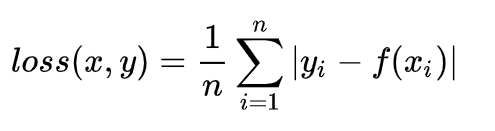其中，yi是真实值，f(xi)是预测值，n是样本点个数

优缺点？

• 优点：无论对于什么样的输入值，都有着稳定的梯度，不会导致梯度爆炸问题，具有较为稳健性的解
• 缺点：在中心点是折点，不能求导，梯度下降时要是恰好学习到w=0就没法接着进行了

什么时候使用？

1. 回归任务
2. 简单模型
3. 神经网络通常比较复杂，直接使用L1 loss作为损失函数的非常少

# L2 loss

L2 loss常用别称：

• L2范数损失
• 最小均方误差（LSE）
• 均方误差（MSE）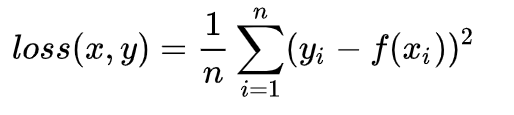其中，yi是真实值，f(xi)是预测值，n是样本点个数

优缺点？

• 优点：各点都连续光滑，方便求导，具有较为稳定的解
• 缺点：不是特别的稳健，因为当函数的输入值距离真实值较远的时候，对应loss值很大在两侧，则使用梯度下降法求解的时候梯度很大，可能导致梯度爆炸

什么时候使用？

1. 回归任务
2. 数值特征不大（防止loss太大，继而引起梯度大，梯度爆炸）
3. 问题维度不高（loss本身比较简单，高纬度的还是得要更复杂的loss支撑）

# Smooth L1 loss

平滑版的L1 loss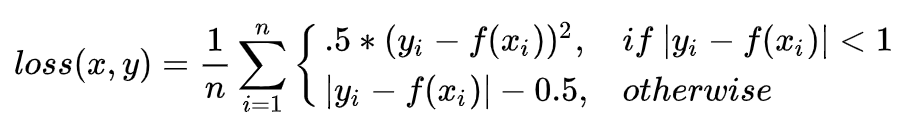分析一下，当预测值f(xi)和真实值yi差别较小的时候（绝对值差小于1），其实使用的是L2 loss；差别大的时候，使用的是L1 loss的平移。因此，Smooth L1 loss其实是L1 loss 和L2 loss的结合，同时拥有两者的部分优点：

1. 真实值和预测值差别较小时（绝对值差小于1），梯度也会比较小（损失函数比普通L1 loss在此处更圆滑）
2. 真实值和预测值差别较大时，梯度值足够小（普通L2 loss在这种位置梯度值就很大，容易梯度爆炸）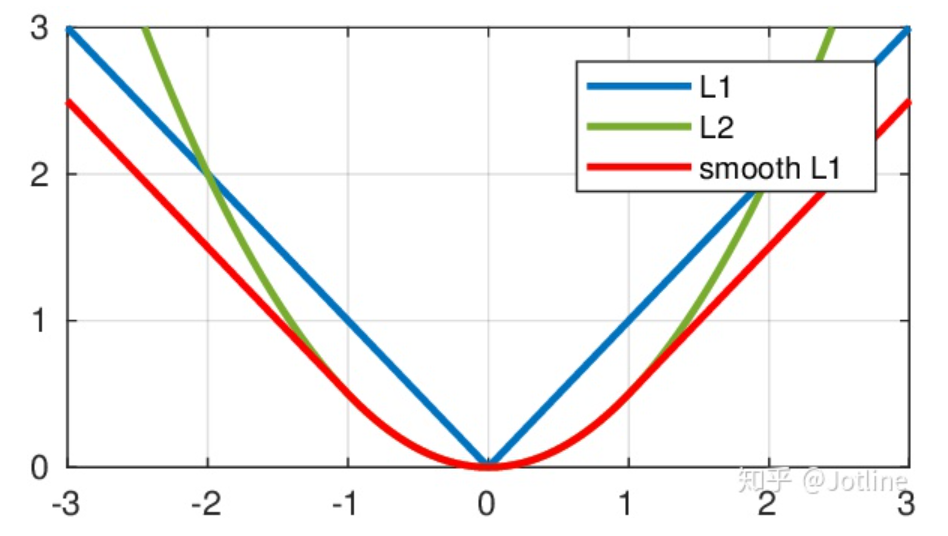什么时候使用？

1. 回归任务
2. 特征中有较大数值
3. 适合大多数问题（用的最多！）

# 三者区别

（1）L1 loss在零点不平滑，此处不可导，所以在w=0时没法接着梯度下降了，用的少
（2）L2 loss对离群点比较敏感，离群点处的梯度很大，容易梯度爆炸
（3）smooth L1 loss结合了L1和L2的优点，修改了零点不平滑问题，且比L2 loss对异常值的鲁棒性更强

# 常见问题解释

## 一、L1 loss、L1正则、L1范数有何区别？

L1范数：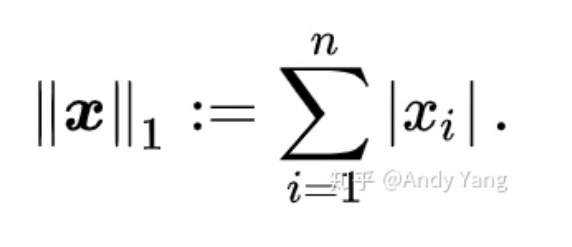L1 loss和L1 正则都使用了L1范数，L1 loss是指对预测值和真实值的差值使用L1范数计算生成的一个损失函数；L1正则是指损失函数之后单独加了一项，这一项是对所有的权重参数w进行L1范数的运算。

两者只是使用了同一种运算方式，但并不是同一个东西，L2同理（注意，L2正则项是L2范数的平方）。

## 二、L1正则为何会引起特征稀疏？

因为L1正则会使得大部分无用特征的权重被置0，这源于公式特性，解释如下：

（1）这是一个L2 loss加上一个L1正则或L2正则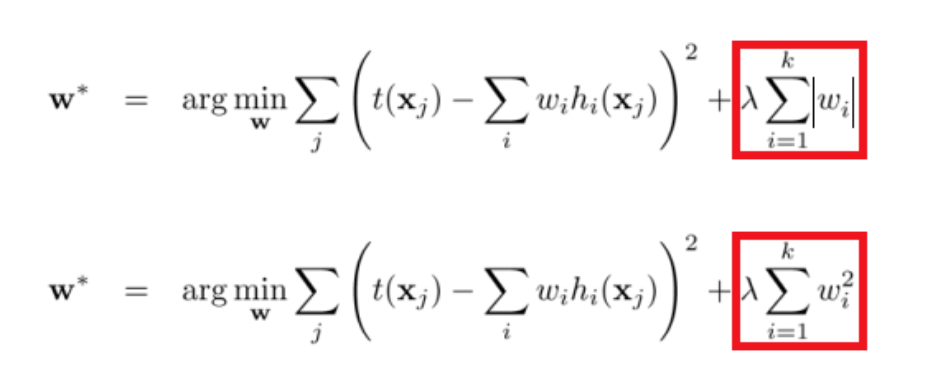（2）梯度下降法优化时，会这样更新参数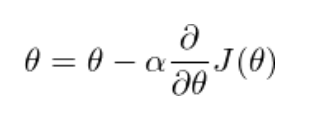（3）对参数w求导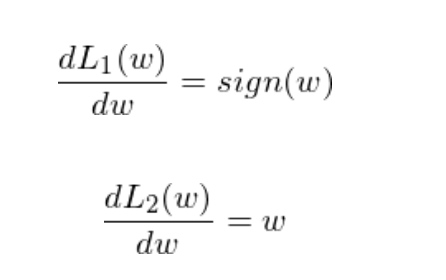（4）下面是L1正则、L2正则的原函数图像和求导之后的函数图像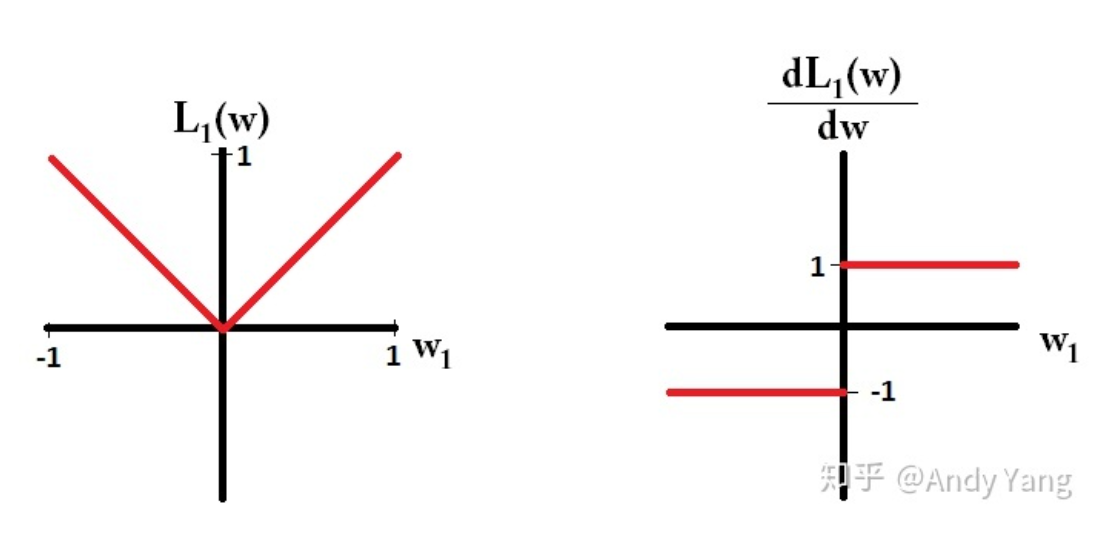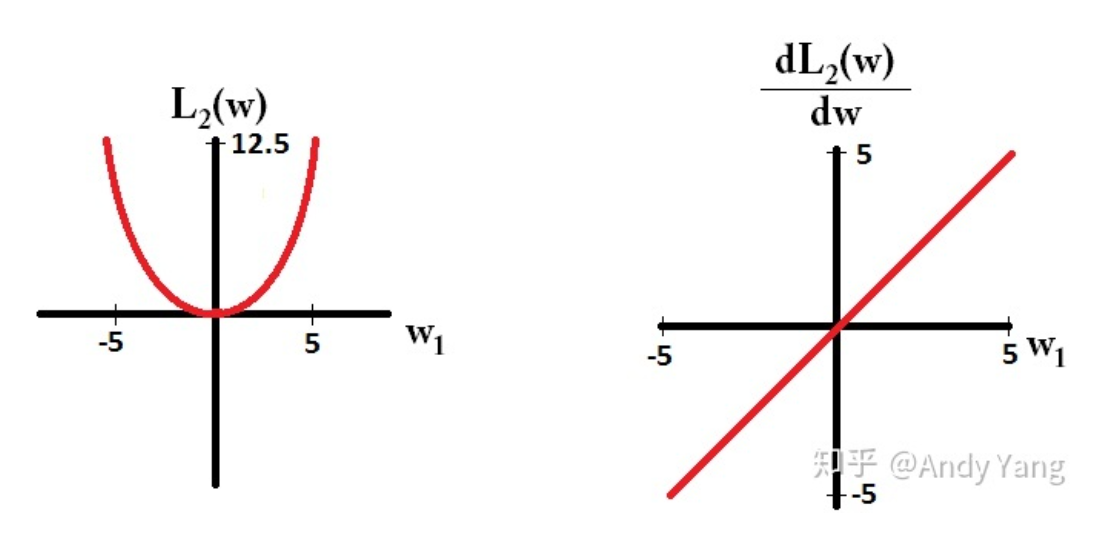（5）先看L1的，在梯度更新时，无论L1的大小是多少，梯度都是1或者-1，所以每次更新时，它都是固定步长向0前进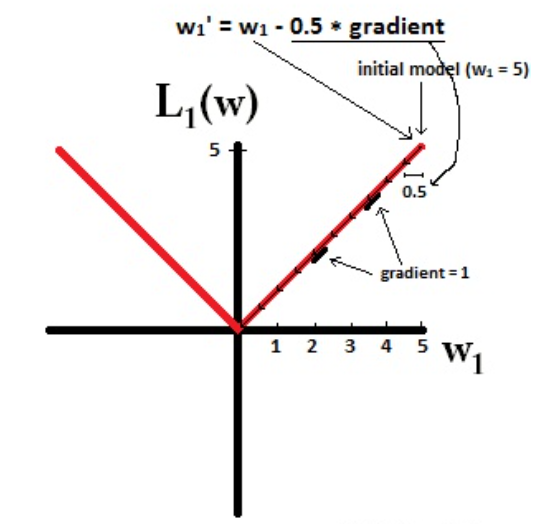（6）再看L2，梯度越靠近0，就会越小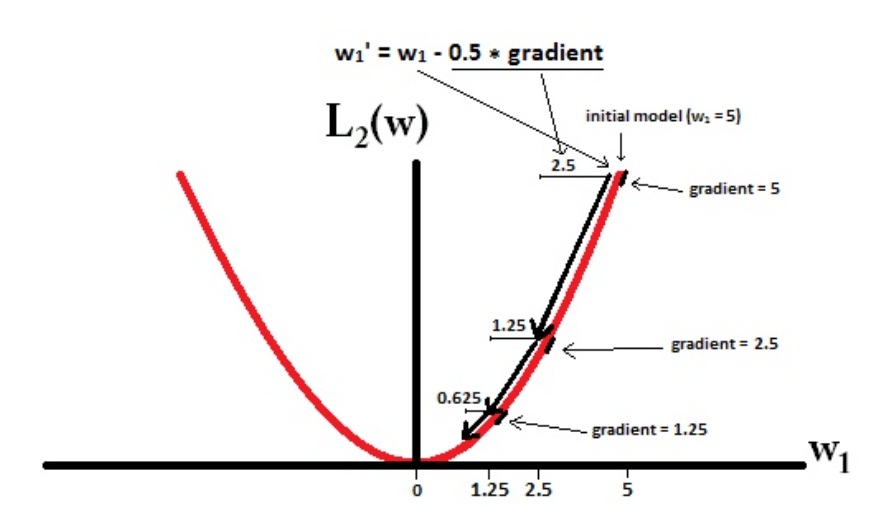（7）也就是说加了L1正则后，经过一定学习步数后w是有可能变为0的，而L2不可能，因为w = w - αw，每次都是减去的原始w的一个比例，永远不会减到0。L1正则中由于很多无用的w可能变为0，则乘上对应位置特征后，对应位置特征也变成0。

因此说L1会造成特征稀疏！

展开全文机器学习 损失函数
• L1 loss & L2 loss & Smooth L1 loss 微信公众号：幼儿园的学霸 个人的学习笔记，关于OpenCV,关于机器学习, …。问题或建议，请公众号留言; 关于神经网络中L1 loss & L2 loss & Smooth L1 loss...

# L1 loss & L2 loss & Smooth L1 loss

微信公众号：幼儿园的学霸
个人的学习笔记，关于OpenCV,关于机器学习, …。问题或建议，请公众号留言;

关于神经网络中L1 loss & L2 loss & Smooth L1 loss损失函数的对比、优缺点分析

# 目录

损失函数曲线如图所示：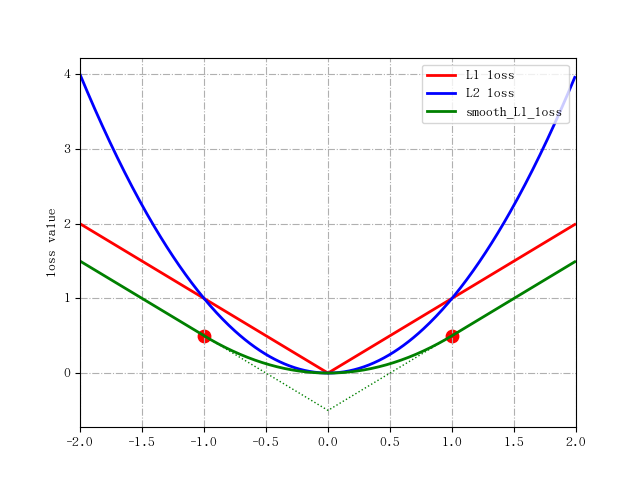# L1 Loss

也就是L1 Loss了，它有几个别称：

• L1 范数损失
• 最小绝对值误差（LAE，LeastAbsolute error）
最常看到的MAE也是指L1 Loss损失函数。 它是把期望值Y 与模型输出值（预测值）f(x)的差 做绝对值得到的误差（添加了绝对值之后意味着该误差只用考虑误差幅度，不用考虑误差的方向，如果未加绝对值，意味着误差是考虑方向的误差，这是另外一种损失）。表示形式及导数如下：

L 1 = ∣ f ( x ) − Y ∣ L 1 ′ = ± f ′ ( x ) (1) L_1=|f(x)-Y| \\ L^{'}_1=\pm f^{'}(x) \tag{1}
对其进行求和，即为总的loss:

L 1 = ∑ i n ∣ f ( x i ) − Y i ∣ (2) L_1 = \sum_i^{n} |f(x_i)-Y_i| \tag{2}

本文为简单，后面的描述未采用求和的形式进行表示。

优点：无论对于什么样的输入值，都有着稳定的梯度，不会导致梯度爆炸问题，具有较为稳健的解
缺点：在中心点是折点，不能求导，不方便求解，可能影响收敛

# L2 Loss

也就是L2 Loss了，它有几个别称：

• L2 范数损失
• 最小均方值偏差（LSD）
• 最小均方值误差（LSE）
最常看到的MSE也是指L2 Loss损失函数,区别在于MSE对loss进行了平均（mean,1/n）操作

它是把期望值Y 与模型输出值（预测值）f(x)求差然后平方得到的误差。表示形式及导数如下：
L 2 = ∣ f ( x ) − Y ∣ 2 L 2 ′ = 2 f ′ ( x ) f ( x ) (3) L_2=|f(x)-Y|^2 \\ L^{'}_2=2f^{'}(x)f(x) \tag{3}
观察图1中L2 loss的误差分布曲线，其中最小值为预测值等于目标值的位置。可以看到，随着误差的增加，损失函数增加的更为迅猛。
优点：各点都连续光滑，方便求导，具有较为稳定的解
缺点：不是特别的稳定（因为当模型输出与期望值相差较大的时候，使用梯度下降法求解的时候梯度很大，可能导致梯度爆炸）

# L1 loss 和L2 loss比较

如下表1所示：

L2损失函数L1损失函数
不是非常的鲁棒(robust)鲁棒
稳定解不稳定解

所谓“鲁棒性”，是指控制系统在一定（结构，大小）的参数摄动下，维持其它某些性能的特性。
所谓“稳定性”，是指控制系统在使它偏离平衡状态的扰动作用消失后，返回原来平衡状态的能力。

• 鲁棒性的分析：
L1损失函数采用的方法之所以是鲁棒的，是因为它能处理数据中的异常值。从直观上说，因为L2范数将误差平方化（如果误差大于1，由于平方作用，误差会被放大很多），模型的误差会比L1范数来得大，因此模型会对这个样本更加敏感，这就需要调整模型来最小化误差。如果这个样本是一个异常值，模型就需要调整以适应单个的异常值（模型会赋予异常点更大的权重），这会牺牲许多其它正常的样本，因为这些正常样本的误差比这单个的异常值的误差小，这样模型会以牺牲其他样本的误差为代价，朝着减小异常点误差的方向更新，这将降低模型的整体性能。

• 稳定性的分析：
该原因来源于L1 loss更新的梯度始终相同，也就是说，即使对于很小的损失值，梯度也很大。这样不利于模型的学习。L2 loss在这种情况下的表现就很好，即便使用固定的学习率也可以有效收敛。L2 loss的梯度随损失增大而增大，而损失趋于0时则会减小。这使得在训练结束时，使用L2 loss模型的结果会更精确。如下图所示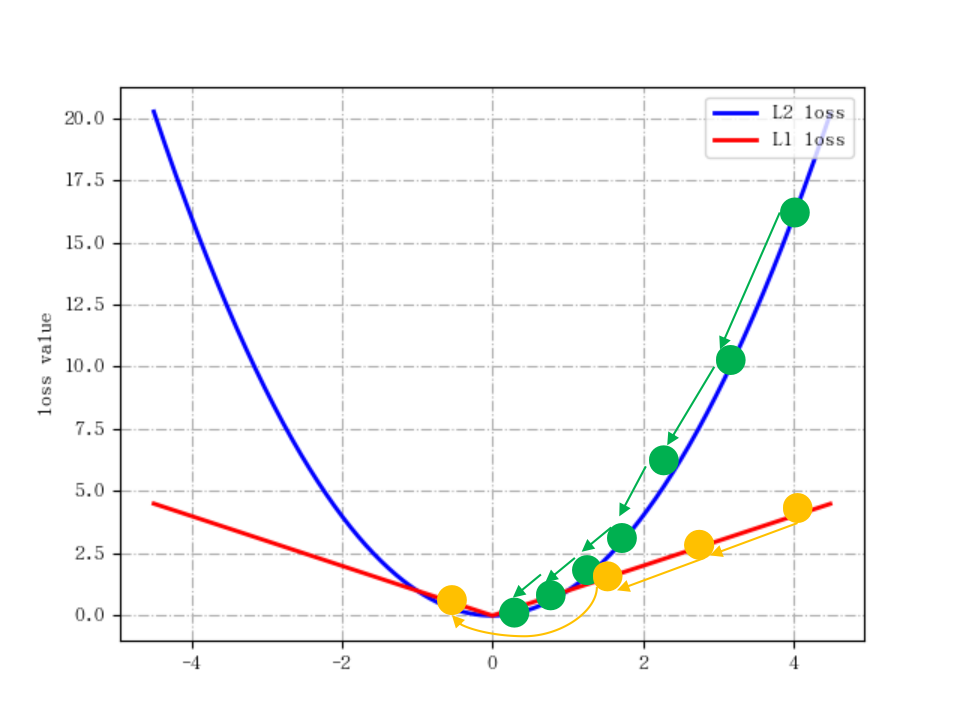L1损失函数采用的方法不稳定性意味着，对于数据集的一个小的水平方向的波动，回归线也许会跳跃很大。在一些数据结构（data configurations）上，该方法有许多连续解；但是，对数据集的一个微小移动，就会跳过某个数据结构在一定区域内的许多连续解。（The method has continuous solutions for some data configurations; however, by moving a datum a small amount, one could “jump past” a configuration which has multiple solutions that span a region. ）在跳过这个区域内的解后，L1 loss偏差线可能会比之前的线有更大的倾斜。相反地，L2 loss的解是稳定的，因为对于一个数据点的任何微小波动，回归线总是只会发生轻微移动；也就说，回归参数是数据集的连续函数。

为了解决L1 loss不稳定这个缺陷，我们可以使用变化的学习率，在损失接近最小值时降低学习率

• 选择：
对于大多数CNN网络，我们一般是使用L2-loss而不是L1-loss，因为L2-loss的收敛速度要比L1-loss要快得多。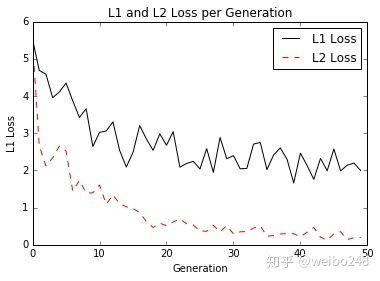对于边框预测回归问题，通常也可以选择平方损失函数（L2损失），但L2范数的缺点是当存在离群点（outliers)的时候，这些点会占loss的主要组成部分。比如说真实值为1，预测10次，有一次预测值为1000，其余次的预测值为1左右，显然loss值主要由1000主宰。所以FastRCNN采用稍微缓和一点绝对损失函数（smooth L1损失），它是随着误差线性增长，而不是平方增长。

• 共同存在的问题：
二者兼有的问题是：在某些情况下，上述两种损失函数都不能满足需求。例如，若数据中90%的样本对应的目标值为150，剩下10%在0到30之间。那么使用L1 loss的模型可能会忽视10%的异常点，而对所有样本的预测值都为150。
这是因为模型会按中位数来预测。而使用L2 loss的模型则会给出很多介于0到30的预测值，因为模型会向异常点偏移。

# Smooth L1 Loss

简单来说就是平滑版的L1 Loss。
smooth L1 loss和L1 loss函数的区别在于，L1 loss在0点处导数不唯一，可能影响收敛。smooth L1 loss的解决办法是在0点附近使用平方函数使得它更加平滑。
函数表达式及导数如下：
s m o o t h   L 1 = { 0.5 ∗ x 2 i f ∣ x ∣ < 1 ∣ x ∣ − 0.5 o t h e r w i s e s m o o t h   L 1 ′ = { x i f ∣ x ∣ < 1 − 1 x < − 1 1 x > 1 (4) smooth \ L_1= \begin{cases} 0.5*x^2 & {if |x|<1}\\ |x|-0.5 & {otherwise} \end{cases} \\ smooth \ L^{'}_1= \begin{cases} x & {if |x|<1}\\ -1 & {x<-1} \\ 1 & {x>1} \end{cases} \tag{4}
损失函数曲线见图1.
仔细观察可以看到，当预测值和ground truth差别较小的时候（绝对值差小于1），其实使用的是L2 Loss；而当差别大的时候，是L1 Loss的平移。Soooth L1 Loss其实是L2Loss和L1Loss的结合，它同时拥有L2 Loss和L1 Loss的部分优点。

• 当预测值和ground truth差别较小的时候（绝对值差小于1），梯度不至于太大。（损失函数相较L1 Loss比较圆滑）
• 当差别大的时候，梯度值足够小（较稳定，不容易梯度爆炸，解决了离群点的梯度爆炸问题）。

# 总结

对于L2 Loss： 当 x 增大时 L2 损失对 x 的导数也增大。这就导致训练初期，预测值与 groud truth 差异过于大时，损失函数对预测值的梯度十分大，训练不稳定。从 L2 Loss的梯度包含 (f(x) - Y)，当预测值 f(x) 与目标值 Y 相差很大时（此时可能是离群点、异常值(outliers)），容易产生梯度爆炸

对于L1 Loss： 根据方程 (1)，L1 对 x 的导数为常数。这就导致训练后期，预测值与 ground truth 差异很小时， L1 损失对预测值的导数的绝对值仍然为 1，而 learning rate 如果不变，损失函数将在稳定值附近波动，难以继续收敛以达到更高精度

对 smooth L1 loss： smooth L1 在x较小时，对x的梯度也会变小，而在x很大时，对x的梯度的绝对值达到上限 1，也不会太大以至于破坏网络参数。smooth L1 loss在 |x| >1的部分采用了 L1 loss，当预测值和目标值差值很大时, 原先L2梯度里的 (f(x) - Y) 被替换成了 ±1,，这样就避免了梯度爆炸, 也就是它更加健壮。smooth L1 完美地避开了 L1 和 L2 损失的缺陷。

smooth L1 loss让loss function对于离群点更加鲁棒，即：相比于L2损失函数，其对离群点/异常值（outlier）不敏感，梯度变化相对更小，训练时不容易跑飞

# 附：图1绘图代码

#!/usr/bin/env python3
#coding=utf-8

#============================#
#Program:plotFunc.py
#
#Date:19-12-3
#Author:liheng
#Version:V1.0
#============================#

import matplotlib.pyplot as plt
import numpy as np

# import matplotlib.font_manager as fm
# # 使用Matplotlib的字体管理器加载中文字体
# my_font=fm.FontProperties(fname="C:\Windows\Fonts\simkai.ttf")

# 支持中文
from pylab import mpl
mpl.rcParams['font.sans-serif'] = ['AR PL UKai CN'] #matplotlib中自带的中文字体
#解决负号'-'显示为方块的问题
plt.rcParams['axes.unicode_minus']=False

x_data = np.arange(-2.0,2.0,0.01)

L1_loss = np.abs(x_data)
L2_loss = np.square(x_data)
smooth_L1_loss = 0.5*np.square(x_data)*(np.abs(x_data)<1) + (np.abs(x_data)-0.5)*(np.abs(x_data)>=1)

x_data_ex = np.arange(-1.0,1.0,0.01)
smooth_L1_loss_ext = (np.abs(x_data_ex)-0.5)*(np.abs(x_data_ex)<=1)

# 指定折线的颜色、线宽和样式
ln2, = plt.plot(x_data, L2_loss, color = 'blue', linewidth = 2.0, linestyle = '-',label='L2 loss')
ln1, = plt.plot(x_data, L1_loss, color = 'red', linewidth = 2.0, linestyle = '-',label='L1 loss')
ln3, = plt.plot(x_data, smooth_L1_loss, color = 'green', linewidth = 2.0, linestyle = '-',label='smooth_L1_loss')
ln4, = plt.plot(x_data_ex, smooth_L1_loss_ext, color = 'green', linewidth = 1.0, linestyle = ':')

plt.xlim([-2,2])
plt.ylabel('loss value')
plt.grid(linestyle='-.')

plt.scatter(1,0.5,s=80,color='red')
plt.scatter(-1,0.5,s=80,color='red')

# 调用legend函数设置图例
# plt.legend(loc='lower right', prop=my_font)
plt.legend(loc='upper right')
# 调用show()函数显示图形
plt.show()

# from matplotlib.font_manager import FontManager
# import subprocess
#
# mpl_fonts = set(f.name for f in FontManager().ttflist)
# print('all font list get from matplotlib.font_manager:')
# for f in sorted(mpl_fonts):
#     print('\t' + f)
# output = subprocess.check_output('fc-list :lang=zh -f "%{family}\n"', shell=True, encoding="utf8")
# zh_fonts = set(f.split(',', 1) for f in output.split('\n'))
#
# print('\n' + 'Chinese font list get from fc-list:')
# for f in sorted(zh_fonts):
#     print('\t' + f)
# print('\n' + 'the fonts we can use:')
# available = set(mpl_fonts) & set(zh_fonts)
# for f in available:
#     print('\t' + f)


# 参考资料

下面的是我的公众号二维码图片，欢迎关注。展开全文• 通常情况下，我们一般使用L2loss，因为L2loss的收敛速度要比L1loss快很多。 L2loss的缺点是在训练过程中存在离群点时，这些点占据loss的主要部分，从而造成训练波动甚至失败。 L1loss在0点处导数不唯一，可能影响...

通常情况下，我们一般使用L2loss，因为L2loss的收敛速度要比L1loss快很多。

L2loss的缺点是在训练过程中存在离群点时，这些点占据loss的主要部分，从而造成训练波动甚至失败。

L1loss在0点处导数不唯一，可能影响模型训练时收敛。

smooth L1loss在较大的范围里使用较为缓和的线性增长，来避免L2loss的问题，而在0点处使用平方函数来使其更加平滑，训练收敛更加稳定，更容易收敛到局部最优，而不会跳出局部最优。

接下来我们看一下其各自的公式：展开全文深度学习 机器学习 神经网络 L2loss
• 文章目录0 前言：1 定义2 特点3 常见的经典误差函数3.1 平均绝对误差MAE(L1 Loss)公式：图像：优点缺点应用场景：Ｐytorch示例：3.2 均方误差MSE（L2 Loss）公式：图像：优点缺点应用场景：pytorch示例：3.3 Smooth ...
•pytorch
• L1 loss 均绝对误差函数（MEAN Absolute Error） 描述模型预测值和真实值之间距离的均值 MAE连续，但是在y-ƒ(x)=0处不可导。并且可以看到MAE的梯度为±1，对于函数的极小值其附近梯度不变，这非常不利于...机器学习 深度学习 神经网络
• ## torch.nn.L1Loss用法

万次阅读 2020-12-21 14:05:45
L1LOSS CLASS torch.nn.L1Loss(size_average=None,reduce=None,reduction: str = 'mean') 创建一个标准来测量输入x和目标y中每个元素之间的平均绝对误差（MAE）。 未减少的损失（即reduction设置为'none'）可以...pytorch
• 常用lossL1： 公式：L1=∑i=1n∣yi−f(xi)∣L1=\sum_{i=1}^{n}\left|y_{i}-f\left(x_{i}\right)\right|L1=i=1∑n​∣yi​−f(xi​)∣ 导数：dL1(x)dx={1 if x≥0−1 otherwise \frac{\...深度学习 机器学习
• 总结对比下$$L_1$$ 损失...均方误差MSE ($$L_2$$ Loss) 均方误差（Mean Square Error,MSE）是模型预测值$$f(x)$$ 与真实样本值$$y$$ 之间差值平方的平均值，其公式如下\[ MSE = \frac{\sum_{i=1}^n(f_{x_i} - y_i...
• 1. L1 loss： 公式和求导公式： （带绝对值求导时，先去掉绝对值符号，再分情况求导） 一个batch的形式： L1 loss 图形和求导图形如下： 图的底部是预测值和label的差值。 我们可以看到L1 loss的底部是...
• 这个博客让我看明白了三者的区别： ... 总结就是smooth L1 loss完美的规避了L1 loss和L2 loss的缺点 相对于L1 loss来说，收敛的更快了 相对于L2 loss来说，对于离群点更加友好，梯度变化小 ...深度学习 神经网络
• 的P23讲讲述了L1Loss函数的使用。 import torch from torch.nn import L1Loss inputs = torch.tensor([1, 2, 3], dtype=torch.float32) # 变成浮点数 targets = torch.tensor([1, 2, 5], dtype=torch.float32) ...pytorch 深度学习 神经网络
• 损失函数是深度学习模型优化的一个灵魂基础，所以无论是很新的transform模型，还是比较早期的AlexNet，都不可避免的要涉及到损失函数的设计和应用。 所以，各种形形色色的损失函数（Loss）也就成了面试...1. L1 loss人工智能 深度学习 机器学习 正则化
• ## L1, L2以及smooth L1loss

万次阅读 2019-06-10 09:48:26
在机器学习实践中，你也许需要在神秘的L1和L2中做出选择。通常的两个决策为：1) L1范数 vs L2范数 的损失函数； 2) L1正则化 vs L2正则化。 作为损失函数   L1范数损失函数，也被称为最小绝对值偏差（LAD），...
• loss = nn.L1Loss() a=torch.FloatTensor([1,2,3]) b=torch.FloatTensor([1.1,2.2,2.9]) output = loss(a, b) print(output) c=torch.FloatTensor([[1,3],[2,3],[1,1]]) d=torch.FloatTensor([[2,3.2],[2.1,3.2],...pytorch 深度学习
• L1 loss和L2 loss的区别？ L1 loss: L2 loss: smooth L1 loss: l1 loss在零点不平滑，用的较少。一般来说，l1正则会制造稀疏的特征，大部分无用的特征的权重会被置为0。 （适合回归任务，简单的模型，由于神经...机器学习
• nn.L1Loss(size_average=True, reduce=False) 2.然后举个简单的例子，帮助理解 1》设置网络的输出与真实的输出： # 其中output为网络的输出 # target为目标输出即对应输入真实的标签 output = torch.ones(2,...pytorch 深度学习
• 讲解的很清楚： https://www.cnblogs.com/wangguchangqing/p/12021638.html深度学习 机器学习 python
• 衡量预测值与真实值的偏差程度的最常见的loss： 误差的L1范数和L2范数 因为L1范数在误差接近0的时候不平滑，所以比较少用到这个范数 对于大多数CNN网络，我们一般是使用L2 loss而不是L1 loss，因为L2 loss的收敛...
• 均方误差MSE (L2 Loss) 均方误差（Mean Square Error，MSE）是模型预测值f(x) 与真实样本值y 之间差值平方的平均值，其公式如下
• 1.20.1.L1Loss（L1范数损失） 1.20.2.MSELoss（均方误差损失） 1.20.3.CrossEntropyLoss （交叉熵损失） 1.20.4.CTCLoss（连接时序分类损失） 1.20.5.NLLLoss（负对数似然损失） 1.20.6.PoissonNLLLoss (目标泊松...
• ## pytorch torch.nn.L1Loss

千次阅读 2020-10-27 21:19:19
应用 # 1)计算绝对差总和：|0-1|+|1-1|+|2-1|+|3-1|=4； # 2)再平均：4/4=1。 import torch import torch.nn as nn ...criterion = nn.L1Loss() loss = criterion(sample, target) API CLASS torch.nn.L1Lopython
• 1、L1Loss原理 L1Loss计算预测值和真实值的mean absolute error (MAE，平均绝对值误差)。 其中： x:(N, *)(N,∗)where*∗means, any number of additional dimensions y:(N, *)(N,∗), same shape as ...pytorch python 计算机视觉
• 本文转载自知乎，已获作者授权转载。链接：https://zhuanlan.zhihu.com/p/342991797目标检测任务的损失函数由Classificition Loss和BBo...算法 深度学习 计算机视觉 人工智能 机器学习
• L1范数误差(L1 loss) 代表：MAE(Mean Absolute Error, 均绝对误差)。即估计值f(x)f(x)f(x)与真实值yyy之间距离的均值。 MAE=1n∑i=1n∣f(xi)−yi∣MAE=\frac{1}{n}\sum_{i=1}^n|f(x_i)-y_i|MAE=n1​i=1∑n​∣f(xi​...神经网络 深度学习
• 平均绝对误差，L1损失 平均绝对误差（MAE）是另一种用于回归模型的损失函数。MAE是目标变量和预测变量之间绝对差值之和。因此它衡量的是一组预测值中的平均误差大小，而不考虑它们的方向（如果我们考虑方向的话，那...回归
• L1范数不可微。但是存在次梯度，即是次微分的 L1正则化相比L2正则化更能得到稀疏解的巧妙解释 - 知乎机器学习 人工智能...